RS Aggarwal Class 9 Solutions Chapter 14 -Statistics Ex 14A (14.1)

RS Aggarwal Class 9 Ex 14A Chapter 14

Q1: The following table shows the number of students participating in various games in a school.

 Game Cricket Football Basketball Tennis No. of students 27 36 18 12

Draw a bar graph to represent the above data.

Sol:

Take the various types of games along the x-axis and the number of students along y-axis.

Along the y-axis, take 1 small square = 3 units.

All the bars should be of the same width and same space should be left between the consecutive bars.

Now, we shall draw the bar chart, as shown below: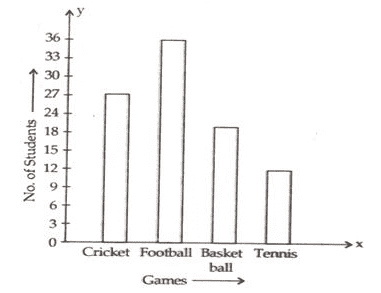Q.2: On a certain day, the temperature in a city was recorded as under:

 Time 5 am 8 am 11 am 3 pm 6 pm Temperature(in Celsius 20 24 26 22 18

Illustrate the data with a bar graph.

Sol:

Take the timings along the x-axis and the temperature along y-axis.

Along the y-axis, take 1 small square = 5 units

All the bars should be of the same width and same space should be left between the consecutive bars.

Now, we shall draw the bar chart, as shown below: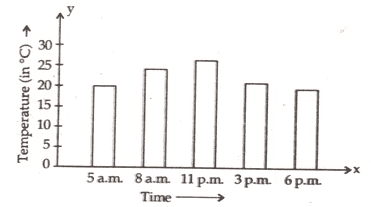Q.3: The approximate velocities of some vehicles are given below:

 Name of vehicles Bicycle Scooter Car Bus Train Velocity(in km/hr) 27 36 18 12 80

Draw a bar graph to represent the above data.

Sol:

Take the mode of transport along the x-axis and the velocities along the y-axis.

Along the y-axis, take 1 small square =10 units

All the bars should be of same width and same space should be left between the consecutive bars.

Now, we shall draw the bar chart, as shown below: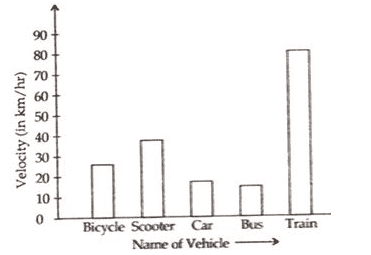Q.4: The following table shows the favorite sports of 250 students of a school. Represent the data by a bar graph.

 Sports Cricket Football Tennis Badminton Swimming No. of students 75 35 50 25 65

Sol:

Take the various types of sports along the x-axis and the number of students along the y-axis.

Along the y-axis, take 1 small square = 10 units

All the bars should be the same width and same space should be left between the consecutive bars.

Now, we shall draw the bar chart, as shown below: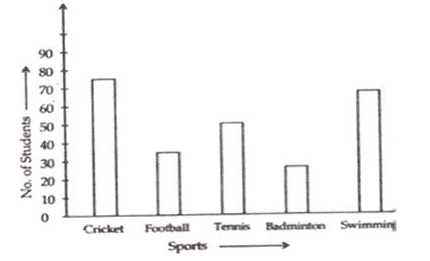Q.5: Given below is the table which shows the year wise strength of a school. Represent this data by a bar graph.

 Year 2001-02 2002-03 2003-04 2004-05 2005-06 No. of students 800 975 1100 1400 1625

Sol:

Take the academic year along the x-axis and the number of students along the y-axis.

Along the y-axis, take 1 big division = 200 units

All the bars should be the same width and same space should be left between the consecutive bars.

Now, we shall draw the bar chart, as shown below: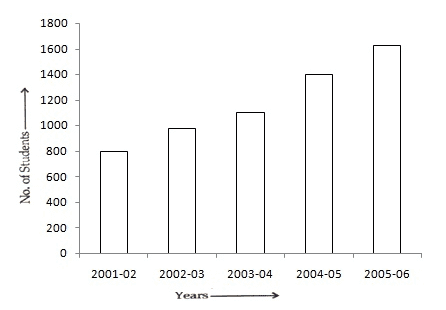Q.6: The following table shows the number of scooters produced by a company during six consecutive years. Draw a bar graph to represent this data.

 Year 1999 2000 2001 2002 2003 2004 No. of Students 11000 14000 12500 17500 15000 24000

Sol:

Take the years along the x-axis and the number of scooters along the y-axis.

Along the y-axis, take 1 big division= 5000 units.

All the bars should be of the same width and same space should be left between the consecutive bars.

Now, we shall draw the bar chart, as shown below: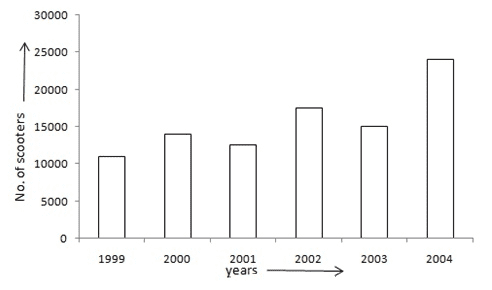Q.7: The birth rate per thousand in five countries over a period of time is shown below:

 Country China India Germany UK Sweden Birth Rate per thousand 42 35 14 28 21

Represent the above data by a bar graph.

Sol:

Take the countries along the x-axis and the birth rate (per thousand) along y-axis.

Along the y-axis, take 1 big division = 5 units

All the bars should be of same width and same space should be left between the consecutive bars.

Now, we shall draw the bar chart, as shown below: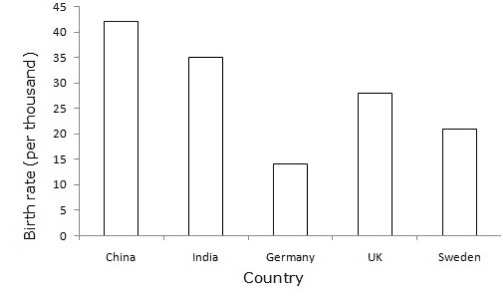Q.8: The following table shows the interest paid by India (in thousand crore rupees) on external debts during the period 1998-99 to 2002-03.

Represent the data by a bar graph.

 Year 1998-99 1999-2000 2000-01 2001-02 2002-03 Interest (in thousand crore rupees) 70 84 98 106 120

Sol:

Take the years along the x-axis and the Interest (in thousand crore rupees) along the y-axis.

Along the y-axis, take 1 big division = 20 units

All the bars should be of the same width and same space should be left between the consecutive bars.

Now, we shall draw the bar chart, as shown below: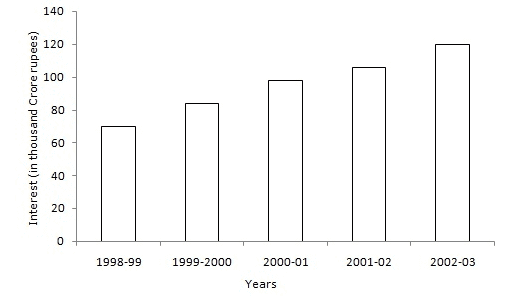Q.9: The air distances of four cities from Delhi (in km) are given below:

 City Kolkata Mumbai Chennai Hyderabad Distance from Delhi( in km) 1340 1100 1700 1220

Draw a bar graph to represent the above data.

Sol:

Take the city along the x-axis and the distance from the Delhi (in km) along the y-axis.

Along the y-axis, take 1 big division= 200 units.

All the bars should be the same width and same space should be left between the consecutive bars.

Now, we shall draw the bar chart, as shown below: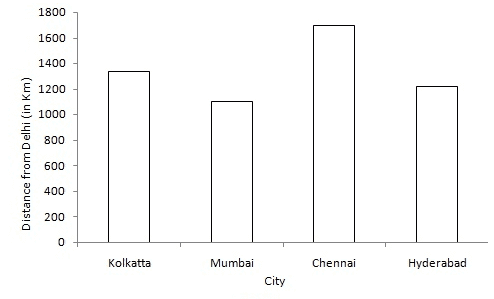Q.10: The following table shows the life expectancy (average age to which people live) in various countries in a particular year.

Represent this data by a bar graph.

 Country Japan India Britain Ethiopia Cambodia Life expectancy(in years) 76 57 70 43 36

Sol:

Take Country along the x-axis and Life expectancy (in years) along they-axis.

Along the y-axis, take 1 big division = 10 units

All the bars should be the same width and same space should be left between the consecutive bars.

Now, we shall draw the bar chart, as shown below: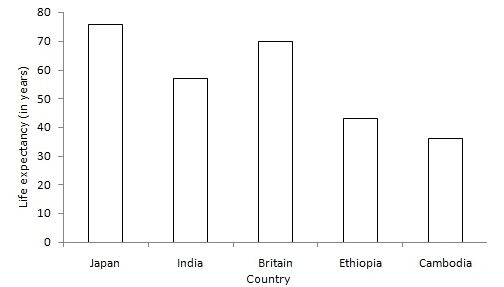Q.11: Gold prices on 4 consecutive Tuesdays were as under:

 Week First Second Third Fourth Rate per 10g (in Rs) 7250 7500 7600 7850

Draw a bar graph to show this information.

Sol:

Take the number of the week along the x-axis and rate per 10 gm ( in Rs.) along the y-axis.

Along the y-axis, take 1 big division = 1000 units

All the bars should be of the same width and same space should be left between the consecutive bars.

Now, we shall draw the bar chart, as shown below: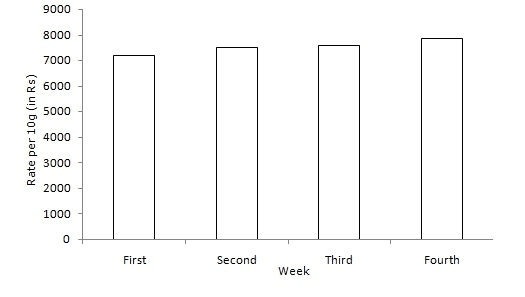Q.12: Various modes of transport used by 1850 students of a school are given below:

 School Bus Private Bus Bicycle Rickshaw By foot 640 360 490 210 150

Draw a bar graph to represent the above data.

Sol:

Take the mode of transport along the x-axis and the number of students along the y-axis.

Along the y-axis, take the 1 big division = 100 units.

All the bars should be the same width and same space should be left between the consecutive bars.

Now, we shall draw the bar chart, case shown below: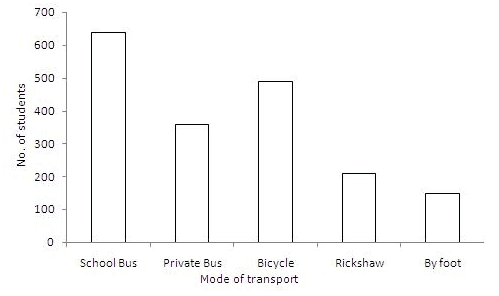Practise This Question

Five beakers A, B, C, D, and E with specific temperatures are shown.